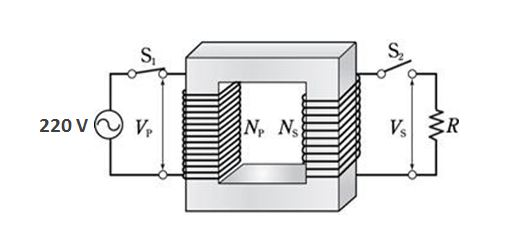# Properties of an electric transformerThe above is a schematic diagram of an electric transformer, where the primary AC voltage is $220\text{ V}$ and the secondary coil is connected with an electric heating instrument. The secondary voltage and current intensity are $110\text{ V}$ and $8\text{ A},$ respectively. Which of the following statements is correct?

There is no energy loss in this electric transformer.

$a)$ The current intensity flowing in the primary winding is $4\text{ A}.$
$b)$ The number of turns in the secondary winding is twice as many as that in the primary winding.
$c)$ The primary coil always transfers $880\text{ W}$ of electric power to the secondary coil without reference to any heating instrument.

×

Problem Loading...

Note Loading...

Set Loading...